Question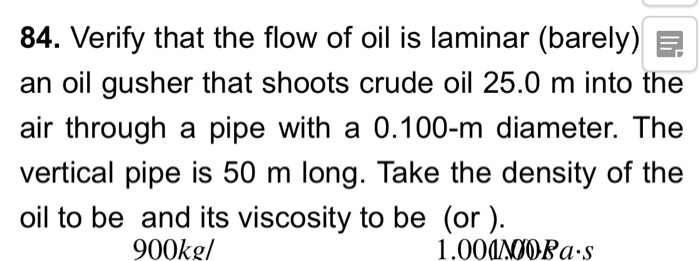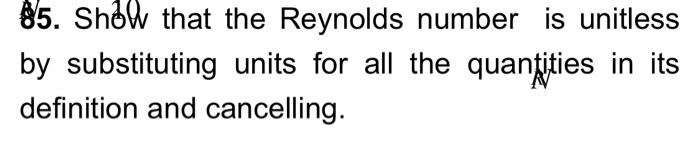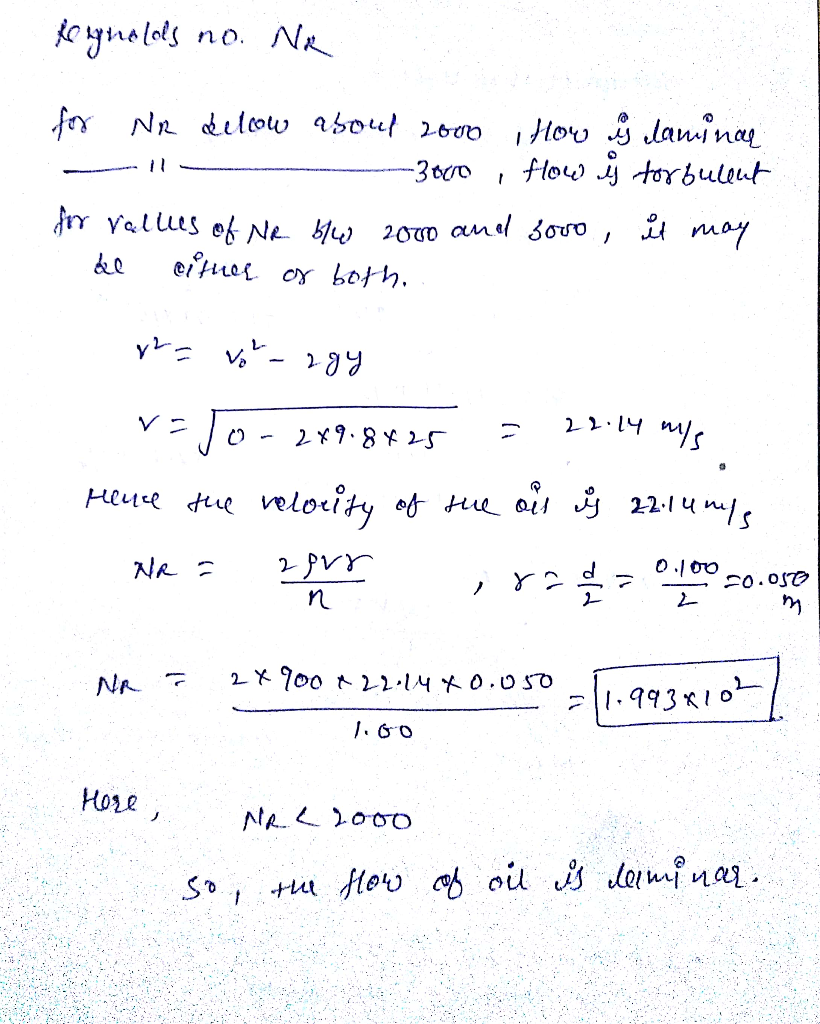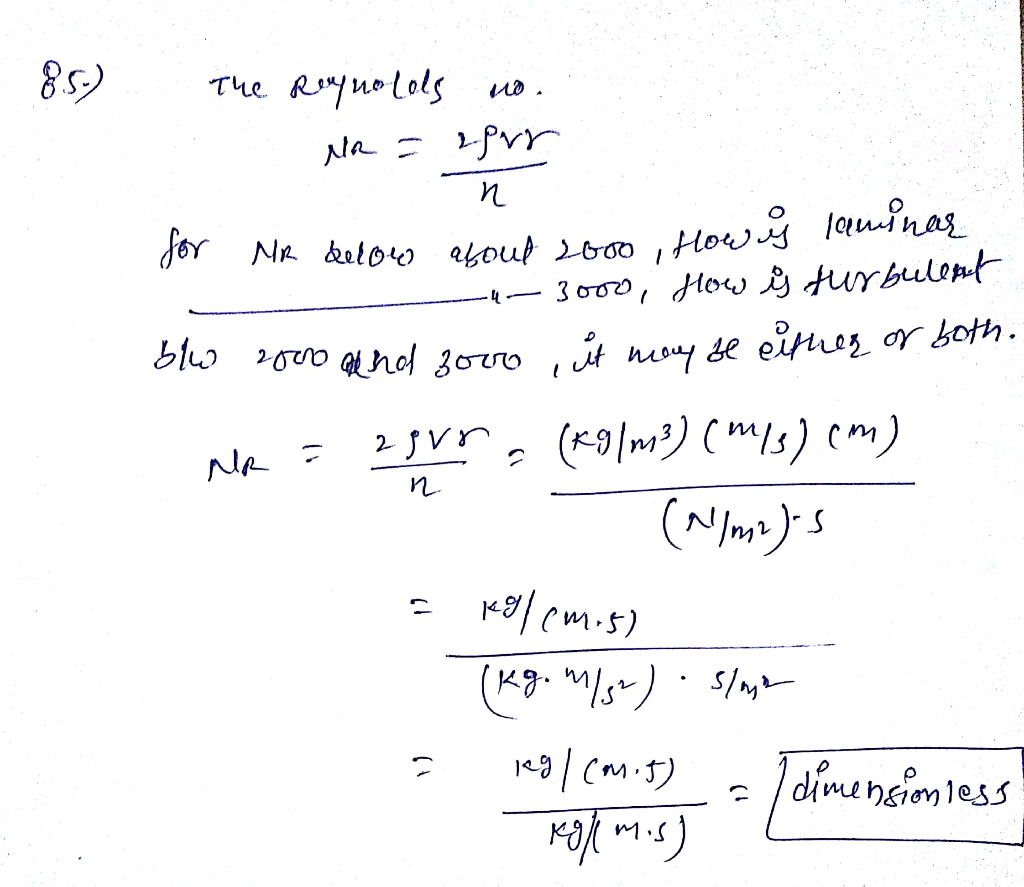#### Earn Coins

Coins can be redeemed for fabulous gifts.

Similar Homework Help Questions
• ### 12.5: The Onset of Turbulence 84. Verify that the flow of oil is laminar (barely) for...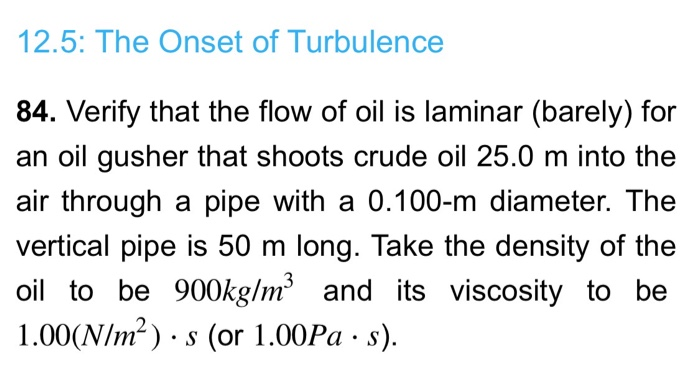12.5: The Onset of Turbulence 84. Verify that the flow of oil is laminar (barely) for an oil gusher that shoots crude oil 25.0 m into the air through a pipe with a 0.100-m diameter. The vertical pipe is 50 m long. Take the density of the oil to be 900kg/m and its viscosity to be 1.00(N/m2s (or 1.00Pa s)

• ### Goal 2 lo introduce you IV The systematic problem-solving techniques CLO2: Use a basic scientific vocabulary...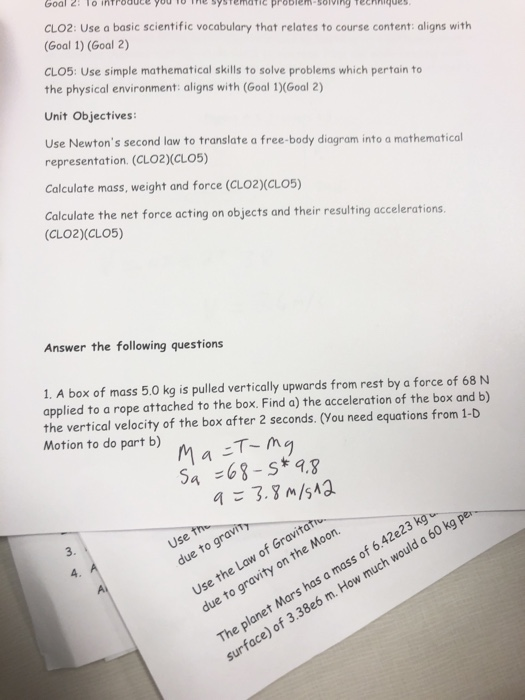Goal 2 lo introduce you IV The systematic problem-solving techniques CLO2: Use a basic scientific vocabulary that relates to course content: aligns with (Goal 1) (Goal 2) CLO5: Use simple mathematical skills to solve problems which pertain to the physical environment: aligns with (Goal 1)(Goal 2) Unit Objectives: Use Newton's second law to translate a free-body diagram into a mathematical representation. (CLO2)(CLO5) Calculate mass, weight and force (CLO2)(CL05) Calculate the net force acting on objects and their resulting accelerations. (CLO2)(CLO5)...

• ### (a) A horizontal pipe of diameter 10 cm carries crude oil from A to C as...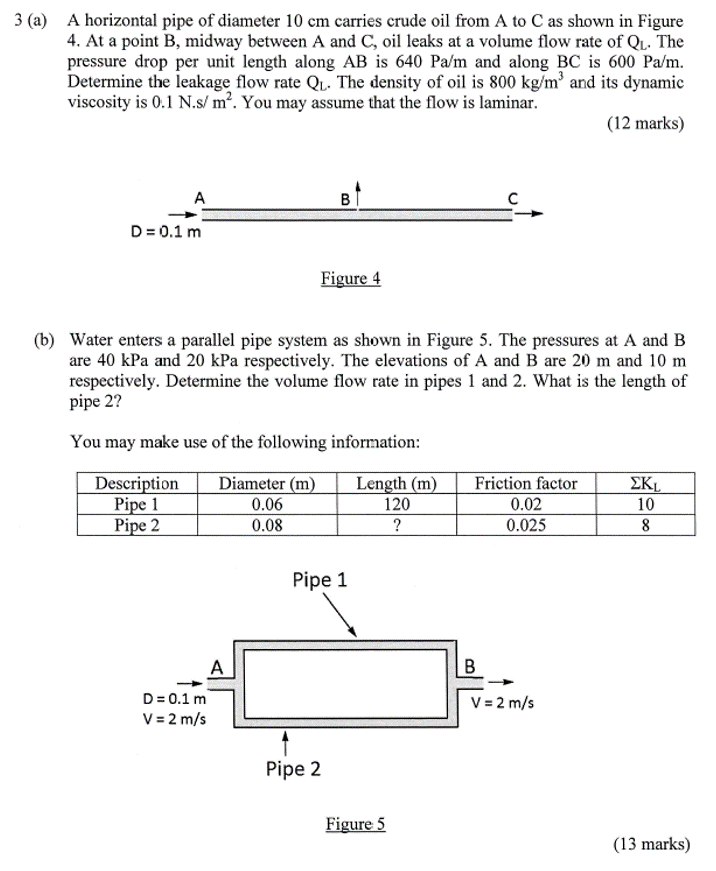(a) A horizontal pipe of diameter 10 cm carries crude oil from A to C as shown in Figure 4. At a point B, midway between A and C, oil leaks at a volume flow rate of QL . The pressure drop per unit length along AB is 640 Pa/m and along BC is 600 Pa/m. Determine the leakage flow rate QL . The density of oil is 800 kg/m3 and its dynamic viscosity is 0.1 N.s/ m2 . You...